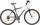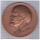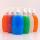# Reciprocal

It is true (prove it) that if a> b> 0:Result

#### Solution:Leave us a comment of example and its solution (i.e. if it is still somewhat unclear...):Be the first to comment!## Next similar examples:

1. Reciprocal equationDetermine the root of the equation: 9/x-7/x=1
2. Eq with reciprocalSolve given equation with reciprocal member: a-6/a+10=4/8
3. Center traverseIt is true that the middle traverse bisects the triangle?
4. Unknown numberIdentify unknown number which 1/5 is 40 greater than one tenth of that number.
5. InequationSolve the inequation: 5k - (7k - 1)≤ 2/5 . (5-k)-2
6. InqualitySolve inequality: 3x + 6 > 14
7. Sum of dividendIf X is divided separately 2.63 and 1.40. And the results of the individual divisions are added to give a result less than X. What is X?
8. Simply equationSolve this equation for x: ?
9. SistersSisters Zuzka Lenka and Vierka has save for a new bike. Zuzka save € 90, Lenka save € 90 and Vierka save € 82. Missing 30% gave them their mother. What is the price of the bike?
10. Linear systemSolve this linear system (two linear equations with two unknowns): x+y =36 19x+22y=720
11. Two numbersWe have two numbers. Their sum is 140. One-fifth of the first number is equal to half the second number. Determine those unknown numbers.
12. BicycleThe bicycle pedal gear has 36 teeth, the rear gear wheel has 10 teeth. How many times turns rear wheel, when pedal wheel turns 120x?
13. Bronze medalTo produce 1 kg of bronze must be 0.95 kg copper, 0.04 kg of tin, 0.01 kg zinc. The bronze medal has a weight of 8.5 g. How much copper, tin and zinc does medal have?
14. Liquid soapLiquid soap lasted two weeks to one member of the family. How many days will last, if it use: a) two members b) 4 members c) seven members?
15. PercentsHow many percents is 900 greater than the number 750?
16. Highway repairThe highway repair was planned for 15 days. However, it was reduced by 30%. How many days did the repair of the highway last?Calculate how many percent will increase the length of an HTML document, if any ASCII character unnecessarily encoded as hexadecimal HTML entity composed of six characters (ampersand, grid #, x, two hex digits and the semicolon). Ie. space as: &#x20;